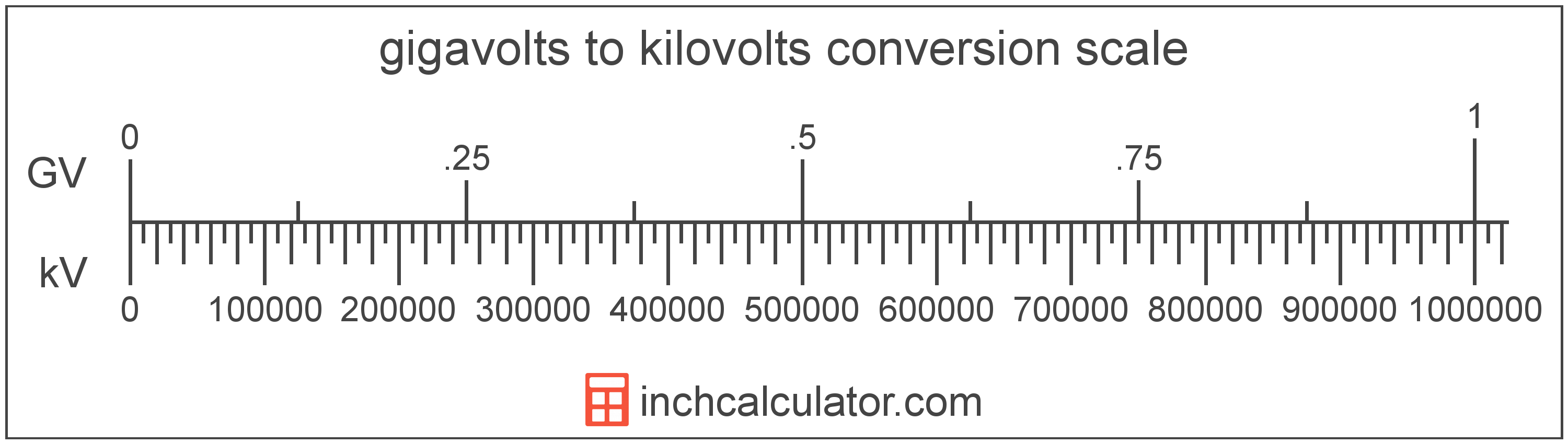# Kilovolts to Gigavolts Conversion

Enter the voltage in kilovolts below to get the value converted to gigavolts.

Results in Gigavolts:1 kV = 1.0E-6 GV
Hint: use a scientific notation calculator to convert E notation to decimal

## How to Convert Kilovolts to Gigavolts

To convert a kilovolt measurement to a gigavolt measurement, divide the voltage by the conversion ratio.

Since one gigavolt is equal to 1,000,000 kilovolts, you can use this simple formula to convert:

gigavolts = kilovolts ÷ 1,000,000

The voltage in gigavolts is equal to the kilovolts divided by 1,000,000.

For example, here's how to convert 5,000,000 kilovolts to gigavolts using the formula above.
5,000,000 kV = (5,000,000 ÷ 1,000,000) = 5 GV## Kilovolts

One kilovolt is equal to 1,000 volts, which are the potential difference that would move one ampere of current against one ohm of resistance.

The kilovolt is a multiple of the volt, which is the SI derived unit for voltage. In the metric system, "kilo" is the prefix for 103. Kilovolts can be abbreviated as kV; for example, 1 kilovolt can be written as 1 kV.

## Gigavolts

One gigavolt is equal to 1,000,000,000 volts, which are the potential difference that would move one ampere of current against one ohm of resistance.

The gigavolt is a multiple of the volt, which is the SI derived unit for voltage. In the metric system, "giga" is the prefix for 109. Gigavolts can be abbreviated as GV; for example, 1 gigavolt can be written as 1 GV.

## Kilovolt to Gigavolt Conversion Table

Kilovolt measurements converted to gigavolts
Kilovolts Gigavolts
1 kV 0.000001 GV
2 kV 0.000002 GV
3 kV 0.000003 GV
4 kV 0.000004 GV
5 kV 0.000005 GV
6 kV 0.000006 GV
7 kV 0.000007 GV
8 kV 0.000008 GV
9 kV 0.000009 GV
10 kV 0.00001 GV
100 kV 0.0001 GV
1,000 kV 0.001 GV
10,000 kV 0.01 GV
100,000 kV 0.1 GV
1,000,000 kV 1 GV